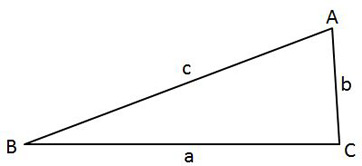# Test Prep Plan - Take a practice test

Take this practice test to check your existing knowledge of the course material. We'll review your answers and create a Test Prep Plan for you based on your results.
How Test Prep Plans work
1
2Based on your results, we'll create a customized Test Prep Plan just for you!
3Study smarter
Study more effectively: skip concepts you already know and focus on what you still need to learn.

# Precalculus: Homework Help Resource Final Exam

Free Practice Test Instructions:

Choose your answer to the question and click 'Continue' to see how you did. Then click 'Next Question' to answer the next question. When you have completed the free practice test, click 'View Results' to see your results. Good luck!

#### Question 2 2. Which of the following is equivalent to the expression below?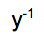#### Question 4 4. Solve the following equation for x.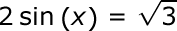#### Question 7 7. What is the equation of the graph below?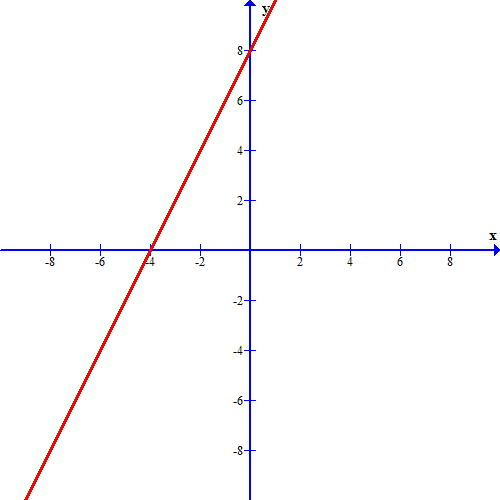#### Question 8 8. The lines a and b are parallel lines of length 5 and 2. The lines d and e are parallel to one another, and both are perpendicular to a and b. What is the length of c?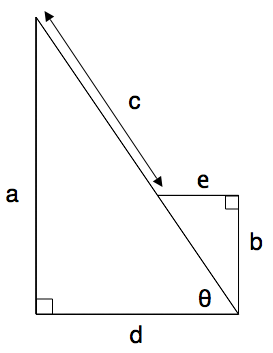#### Question 12 12. What would the first step be in completing the square of the following equation?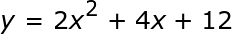#### Question 15 15. In the pictured triangle, ∠A is 62 degrees and ∠C is 86 degrees. If side a is 112 units long, approximately how long is side c?